# What is Star Delta Starter?

Posted on

The most commonly used method for starting of a three-phase induction motor is the star-delta method. This method of starting applies a star delta starter to start an induction motor. This starter facilitates the stator winding to run in delta form and in star form for its beginning state.

Here, the motor starts with a star connected stator winding, and as when motor reached about 80% of its full load speed, it begins to run in delta connected stator winding.

# What is Star Delta Starter?

Star delta starter is a type of reduced voltage starter. We use it to reduce starting current without using any external device or apparatus. The starter mainly consists of a TPDP switch which stands for Tripple Pole Double Throw switch. This switch changes stator winding from star to delta.

During starting condition stator winding is connected in the form of a star. Now we shall see how a star delta starter reduces the starting current of a three-phase induction motor.

For that let us consider,

• VL = Supply Line Voltage,
• ILS = Supply Line Current and,
• IPS = Winding Current per Phase and Z = Impedance per phase winding at stand still condition.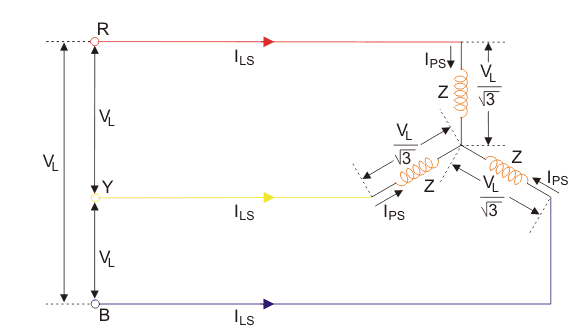As the winding is star connected, the winding current per phase (IPS) equals to supply line current (ILS).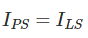As the winding is star connected, the voltage across each phase of the winding is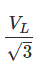Hence, the winding current per phase is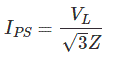Since here, the winding current per phase (IPS) equals to the supply line current (ILS), we can write,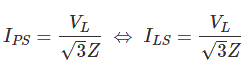Now, let us consider the situation where the motor gets started with delta connected stator winding from same three phase supply points,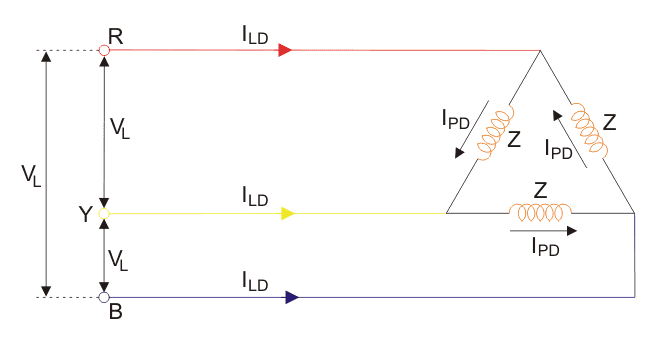Here,

• ILD = Supply Line Current and,
• IPD = Winding Current per Phase and Z = Impedance per phase winding at stand still condition.

As the winding is delta connected, supply line current (ILD) is root three times of the winding current per phase (IPD)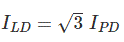As the winding is delta connected, the voltage across each phase of the winding is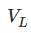Hence, the winding current per phase is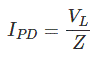Now, we can write,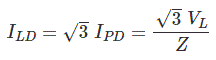Now, by comparing supply line currents drawn by an induction motor with star and delta connected winding, we get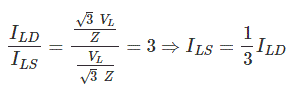Thus we can say that the starting current from the mains in case of star delta is one-third of direct switching in the delta. Again, we know that the starting torque of an induction motor is proportional to the square of the voltage applied to the winding per phase.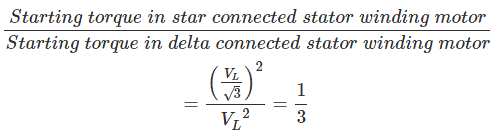The equation shows that star delta starter reduces the starting torque to one-third of that produced by DOL starter. The star-delta starter is equivalent to an autotransformer with a 57.7% tapping.

## Advantages of Star Delta Starter

1. Inexpensive
2. No heat is produced, or tap changing device needs to be used, hence efficiency increases.
3. Starting current reduced to 1/3 of direct online starting current.
4. Produce high torque per ampere of line current.

## Disadvantages of Star Delta Starter

1. Starting torque is reduced to 1/3 of full load torque.
2. A particular set of motors required.

## Applications of Star Delta Starter

By seeing above advantages and disadvantages, we can say that we usually use star delta starter where the required starting current is low and where the line current draw must be at a minimum value. Example – Centrifugal compressor.